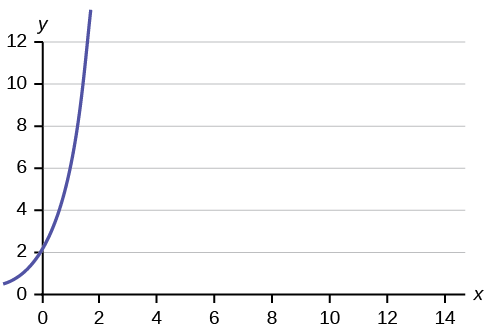# 9.2: Linear Equations

•• OpenStax
• OpenStax
$$\newcommand{\vecs}{\overset { \rightharpoonup} {\mathbf{#1}} }$$ $$\newcommand{\vecd}{\overset{-\!-\!\rightharpoonup}{\vphantom{a}\smash {#1}}}$$$$\newcommand{\id}{\mathrm{id}}$$ $$\newcommand{\Span}{\mathrm{span}}$$ $$\newcommand{\kernel}{\mathrm{null}\,}$$ $$\newcommand{\range}{\mathrm{range}\,}$$ $$\newcommand{\RealPart}{\mathrm{Re}}$$ $$\newcommand{\ImaginaryPart}{\mathrm{Im}}$$ $$\newcommand{\Argument}{\mathrm{Arg}}$$ $$\newcommand{\norm}{\| #1 \|}$$ $$\newcommand{\inner}{\langle #1, #2 \rangle}$$ $$\newcommand{\Span}{\mathrm{span}}$$ $$\newcommand{\id}{\mathrm{id}}$$ $$\newcommand{\Span}{\mathrm{span}}$$ $$\newcommand{\kernel}{\mathrm{null}\,}$$ $$\newcommand{\range}{\mathrm{range}\,}$$ $$\newcommand{\RealPart}{\mathrm{Re}}$$ $$\newcommand{\ImaginaryPart}{\mathrm{Im}}$$ $$\newcommand{\Argument}{\mathrm{Arg}}$$ $$\newcommand{\norm}{\| #1 \|}$$ $$\newcommand{\inner}{\langle #1, #2 \rangle}$$ $$\newcommand{\Span}{\mathrm{span}}$$$$\newcommand{\AA}{\unicode[.8,0]{x212B}}$$

Linear regression for two variables is based on a linear equation with one independent variable. The equation has the form:

$y = a + b\text{x}\nonumber$

where $$a$$ and $$b$$ are constant numbers. The variable $$x$$ is the independent variable, and $$y$$ is the dependent variable. Typically, you choose a value to substitute for the independent variable and then solve for the dependent variable.

##### Example $$\PageIndex{1}$$

The following examples are linear equations.

$y = 3 + 2\text{x}\nonumber$

$y = -0.01 + 1.2\text{x}\nonumber$

##### Exercise $$\PageIndex{1}$$

Is the following an example of a linear equation?

$y = -0.125 - 3.5\text{x}\nonumber$

yes

The graph of a linear equation of the form $$y = a + b\text{x}$$ is a straight line. Any line that is not vertical can be described by this equation.

##### Example $$\PageIndex{2}$$

Graph the equation $$y = -1 + 2\text{x}$$.Figure $$\PageIndex{1}$$.
##### Exercise $$\PageIndex{2}$$

Is the following an example of a linear equation? Why or why not?Figure $$\PageIndex{2}$$.

No, the graph is not a straight line; therefore, it is not a linear equation.

##### Example $$\PageIndex{3}$$

Aaron's Word Processing Service (AWPS) does word processing. The rate for services is $32 per hour plus a$31.50 one-time charge. The total cost to a customer depends on the number of hours it takes to complete the job.

Find the equation that expresses the total cost in terms of the number of hours required to complete the job.

Let $$x =$$ the number of hours it takes to get the job done.

Let $$y =$$ the total cost to the customer.

## Summary

The most basic type of association is a linear association. This type of relationship can be defined algebraically by the equations used, numerically with actual or predicted data values, or graphically from a plotted curve. (Lines are classified as straight curves.) Algebraically, a linear equation typically takes the form $$y = mx + b$$, where $$m$$ and $$b$$ are constants, $$x$$ is the independent variable, $$y$$ is the dependent variable. In a statistical context, a linear equation is written in the form $$y = a + bx$$, where $$a$$ and $$b$$ are the constants. This form is used to help readers distinguish the statistical context from the algebraic context. In the equation $$y = a + b\text{x}$$, the constant b that multiplies the $$x$$ variable ($$b$$ is called a coefficient) is called the slope. The constant a is called the $$y$$-intercept.

The slope of a line is a value that describes the rate of change between the independent and dependent variables. The slope tells us how the dependent variable ($$y$$) changes for every one unit increase in the independent ($$x$$) variable, on average. The $$y$$-intercept is used to describe the dependent variable when the independent variable equals zero.

## Formula Review

$$y = a + b\text{x}$$ where a is the $$y$$-intercept and $$b$$ is the slope. The variable $$x$$ is the independent variable and $$y$$ is the dependent variable.

This page titled 9.2: Linear Equations is shared under a CC BY 4.0 license and was authored, remixed, and/or curated by OpenStax via source content that was edited to the style and standards of the LibreTexts platform; a detailed edit history is available upon request.